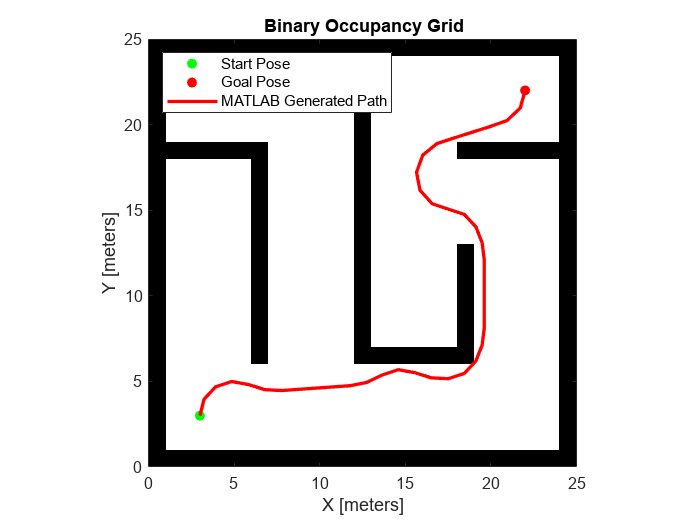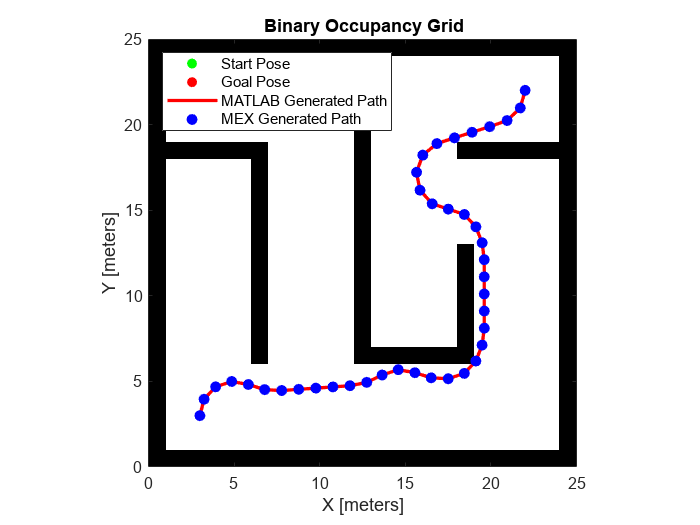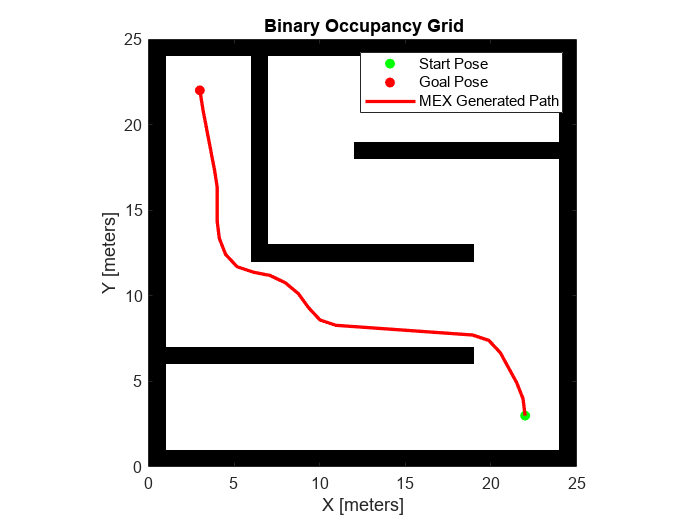# Generate Code for Path Planning Using Hybrid A Star

This example shows how to perform code generation to plan a collision-free path for a vehicle through a map using the Hybrid A* algorithm. After you verify the algorithm in MATLAB®, use the `codegen` (MATLAB Coder) function to generate a MEX function. Use the generated MEX file in the algorithm to visualize the planned path.

### Write Algorithm to Plan Path

Create a function, `codegenPathPlanner`, that uses a `plannerHybridAStar` object to plan a path from the start pose to the goal pose in the map.

```function path = codegenPathPlanner(mapData,startPose,goalPose) %#codegen % Create a binary occupancy map map = binaryOccupancyMap(mapData); % Create a state space object stateSpace = stateSpaceSE2; % Update state space bounds to be the same as map limits. stateSpace.StateBounds = [map.XWorldLimits;map.YWorldLimits;[-pi pi]]; % Construct a state validator object using the statespace and map object validator = validatorOccupancyMap(stateSpace,Map=map); % Set the validation distance for the validator validator.ValidationDistance = 0.01; % Assign the state validator object to the plannerHybridAStar object planner = plannerHybridAStar(validator); % Compute a path for the given start and goal poses pathObj = plan(planner,startPose,goalPose); % Extract the path poses from the path object path = pathObj.States; end ```

This function acts as a wrapper for a standard Hybrid A* path planning call. It accepts standard inputs and returns a collision-free path as an array. Because you cannot use a handle object as an input or output of a function that is supported for code generation, create the planner object inside the function. Save the `codegenPathPlanner` function in your current folder.

### Verify Path Planning Algorithm in MATLAB

Verify the path planning algorithm in MATLAB before generating code.

Generate a random 2-D maze map.

```map = mapMaze(5,MapSize=[25 25],MapResolution=1); mapData = occupancyMatrix(map);```

Define start and goal poses as [x y theta] vectors. x and y specify the position in meters, and theta specifies the orientation angle in radians.

```startPose = [3 3 pi/2]; goalPose = [22 22 pi/2];```

Plan the path for the specified start pose, and goal pose, and map.

`path = codegenPathPlanner(mapData,startPose,goalPose);`

Visualize the computed path.

```show(binaryOccupancyMap(mapData)) hold on % Start state scatter(startPose(1,1),startPose(1,2),"g","filled") % Goal state scatter(goalPose(1,1),goalPose(1,2),"r","filled") % Path plot(path(:,1),path(:,2),"r-",LineWidth=2) legend("Start Pose","Goal Pose","MATLAB Generated Path") legend(Location="northwest")```### Generate Code for Path Planning Algorithm

You can use either the `codegen` (MATLAB Coder) function or the MATLAB Coder (MATLAB Coder) app to generate code. For this example, generate a MEX file by calling `codegen` at the MATLAB command line. Specify sample input arguments for each input to the function using the `-args` option and `func_inputs` input argument.

Call the `codegen` function and specify the input arguments in a cell array. This function creates a separate `codegenPathPlanner_mex` function to use. You can also produce C code by using the `options` input argument. This step can take some time.

`codegen -config:mex codegenPathPlanner -args {mapData,startPose,goalPose}`
```Code generation successful. ```

### Verify Results Using Generated MEX Function

Plan the path by calling the MEX version of the path planning algorithm for the specified start pose, and goal pose, and map.

`mexPath = codegenPathPlanner_mex(mapData,startPose,goalPose);`

Visualize the path computed by the MEX version of the path planning algorithm.

```scatter(mexPath(:,1),mexPath(:,2),... Marker="o",... MarkerFaceColor="b",... MarkerEdgeColor="b") legend("Start Pose","Goal Pose","MATLAB Generated Path","MEX Generated Path") legend(Location="northwest") hold off```### Check Performance of Generated Code

Compare the execution time of the generated MEX function to the execution time of your original function by using `timeit`.

`time = timeit(@() codegenPathPlanner(mapData,startPose,goalPose))`
```time = 0.3735 ```
`mexTime = timeit(@() codegenPathPlanner_mex(mapData,startPose,goalPose))`
```mexTime = 0.0474 ```
`time/mexTime`
```ans = 7.8872 ```

In this example, the MEX function runs more than five times faster. Results may vary for your system.

### Plan Path in New Map Using Generated MEX Function

Plan a path for a new start and goal poses in a new map. The size of the new map must be the same as the map used to generate the MEX function.

Generate a random 2-D maze map.

```mapNew = mapMaze(5,MapSize=[25 25],MapResolution=1); mapDataNew = occupancyMatrix(mapNew);```

Specify start and goal poses.

```startPoseNew = [22 3 pi/2]; goalPoseNew = [3 22 pi/2];```

Plan the path for the specified start pose, and goal pose, and map.

`pathNew = codegenPathPlanner_mex(mapDataNew,startPoseNew,goalPoseNew);`

Visualize the new path computed by the MEX function.

```show(binaryOccupancyMap(mapDataNew)) hold on % Start state scatter(startPoseNew(1,1),startPoseNew(1,2),"g","filled") % Goal state scatter(goalPoseNew(1,1),goalPoseNew(1,2),"r","filled") % Path plot(pathNew(:,1),pathNew(:,2),"r-",LineWidth=2) legend("Start Pose","Goal Pose","MEX Generated Path") legend(Location='northeast')```